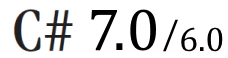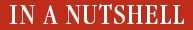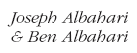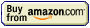# What's new in C# 3.0: Primer

The following extracts from C# 3.0 in a Nutshell explain four of the new features of C# 3.0: Lambda Expressions, Extension Methods, Implicit Typing, and Anonymous Types. These are the core features upon which LINQ queries build.

## Lambda Expressions

A lambda expression is an unnamed method written in place of a delegate instance. The compiler immediately converts the lambda expression to either:

• A delegate instance
• An expression tree, of type Expression<TDelegate>, representing the code inside the lambda expression in a traversable object model. This allows the lambda expression to be interpreted later at runtime.

Given the following delegate type:

`delegate int Transformer (int i);`

we could assign and invoke the lambda expression x => x * x as follows:

```Transformer sqr = x => x * x;
Console.WriteLine (sqr(3));    // 9```

Internally, the compiler resolves lambda expressions of this type writing a private method, and moving the expression’s code into that method.

A lambda expression has the following form:

`(parameters) => expression-or-statement-block`

For convenience, you can omit the parentheses if and only if there is exactly one parameter of an inferable type.
In our example, there is a single parameter, x, and the expression is x * x:

`x => x * x;`

Each parameter of the lambda expression corresponds to a delegate parameter, and the type of the expression (which may be void) corresponds to the return type of the delegate.

In our example, x corresponds to parameter i, and the expression x * x corresponds to the return type int, therefore being compatible with the Transformer delegate:

`delegate int Transformer (int i);`

A lambda expression’s code can be a statement block instead of an expression. We can rewrite our example as follows:

`x => {return x * x;};`

### Explicitly Specifying Lambda Parameter Types

The compiler can usually infer the type of lambda parameters contextually. When this is not the case, you must specify the type of each parameter explicitly. Consider the following delegate type:

`delegate int Transformer (int i);`

The compiler uses type inference to infer that x is an int, by examining Transfomer’s parameter type:

`Transformer d = x => x * x;`

We could explicitly specify x’s type as follows:

`Transformer d = (int x) => x * x;`

### Generic Lambda Expressions and the Func Delegates

With generic delegates, it becomes possible to write a small set of delegate types that are so general they can work for methods of any return type and any (reasonable) number of arguments. These delegates are the Func and Action delegates, defined in the System namespace. Here are the Func delegates (notice that TResult is always the last type parameter):

```delegate TResult Func <T> ();

delegate TResult Func <T, TResult>
(T arg1);

delegate TResult Func <T1, T2, TResult>
(T1 arg1, T2 arg2);

delegate TResult Func <T1, T2, T3, TResult>
(T1 arg1, T2 arg2, T3 arg3);

delegate TResult Func <T1, T2, T3, T4, TResult>
(T1 arg1, T2 arg2, T3 arg3, T4 arg4);```

Here are the Action delegates:

```delegate void Action();

delegate void Action <T>
(T1 arg1);

delegate void Action <T1, T2>
(T1 arg1, T2 arg2);

delegate void Action <T1, T2, T3>
(T1 arg1, T2 arg2, T3 arg3);

delegate void Action <T1, T2, T3, T4>
(T1 arg1, T2 arg2, T3 arg3, T4 arg4);```

These delegates are extremely general. The Transformer delegate in our previous example can be replaced with a Func delegate that takes a single int argument and returns an int value:

```Func<int,int> sqr = x => x * x;
Console.WriteLine (sqr(3));     // 9```

### Outer Variables

A lambda expression can reference the local variables and parameters of the method in which it’s defined. For example:

```static void Main()
{
int factor = 2;
Func<int, int> multiplier = n => n * factor;
Console.WriteLine (multiplier (3));           // 6
}```

Local variables and parameters referenced by a lambda expression are called outer variables or captured variables. A lambda expression that includes outer variables is called a closure.

Outer variables are evaluated when the delegate is actually invoked, not when the variables were captured:

```int factor = 2;
Func<int, int> multiplier = n => n * factor;
factor = 10;
Console.WriteLine (multiplier (2));   // 20```

Lambda expressions can themselves update captured variables:

```int seed = 0;
Func<int> natural = () => seed++;
Console.WriteLine (natural());      // 0
Console.WriteLine (natural());      // 1```

Outer variables have their lifetimes extended to that of the delegate. In the following example, the local variable seed would ordinarily disappear from scope when Natural finished executing. But because seed has been captured, its lifetime is extended to that of the capturing delegate, natural:

```static Func<int> Natural()
{
int seed = 0;
return () => seed++; // Returns a closure
}

static void Main()
{
Func<int> natural = Natural();
Console.WriteLine (natural()); // 0
Console.WriteLine (natural()); // 1
}```

A local variable instantiated within a lambda expression is unique per invocation of the delegate instance. If we refactor our previous example to instantiate seed within the lambda expression, we get a different (in this case, undesirable) result:

```static Func<int> Natural()
{
return() => { int seed = 0; return seed++; };
}

static void Main()
{
NumericSequence natural = Natural();
Console.WriteLine (natural());      // 0
Console.WriteLine (natural());      // 0
}```

## Extension Methods

Extension methods allow an existing type to be extended with new methods, without altering the definition of the original type. An extension method is a static method of a static class, where the this modifier is applied to the first parameter. The type of the first parameter will be the type that is extended. For example:

```public static class StringHelper
{
public static bool IsCapitalized (this string s)
{
if (string.IsNullOrEmpty (s)) return false;
return char.IsUpper (s);
}
}```

The IsCapitalized extension method can be called as though it were an instance method on a string, as follows:

`Console.Write ("Perth".IsCapitalized());`

An extension method call, when compiled, is translated back into an ordinary static method call:

`Console.Write (StringHelper.IsCapitalized ("Perth"));`

Interfaces can be extended, too:

```public static T First<T> (this IEnumerable<T> sequence)
{
foreach (T element in sequence)
return element;

throw new InvalidOperationException ("No elements!");
}
...
Console.WriteLine ("Seattle".First());   // S```

### Extension Method Chaining

Extension methods, like instance methods, provide a tidy way to chain functions. Consider the following two functions:

```public static class StringHelper
{
public static string Pluralize (this string s) {...}
public static string Capitalize (this string s) {...}
}```

x and y are equivalent and both evaluate to "Sausages", but x uses extension methods, whereas y uses static methods:

```string x = "sausage".Pluralize().Capitalize();

string y = StringHelper.Capitalize (StringHelper.Pluralize ("sausage"));```

### Ambiguity and Resolution

#### Namespaces

An extension method cannot be accessed unless the namespace is in scope (typically imported with a using statement.)

#### Extension methods versus instance methods

Any compatible instance method will always take precedence over an extension method. In the following example, Test’s Foo method will always take precedence—even when called with an argument x of type int:

```class Test
{
public void Foo (object x) { }    // This method always wins
}

static class Extensions
{
public static void Foo (this Test t, int x) { }
}```

The only way to call the extension method in this case is via normal static syntax; in other words, Extensions.Foo(…).

#### Extension methods versus extension methods

If two extension methods have the same signature, the extension method must be called as an ordinary static method to disambiguate the method to call. If one extension method has more specific arguments, however, the more specific method takes precedence.

To illustrate, consider the following two classes:

```static class StringHelper
{
public static bool IsCapitalized (this string s) {...}
}

static class ObjectHelper
{
public static bool IsCapitalized (this object s) {...}
}```

The following code calls StringHelper’s IsCapitalized method:

`bool test1 = "Perth".IsCapitalized();`

To call ObjectHelper’s IsCapitalized method, we must specify it explicitly:

`bool test2 = (ObjectHelper.IsCapitalized ("Perth"));`

## var—Implicitly Typed Local Variables

It is often the case that you declare and initialize a variable in one step. If the compiler is able to infer the type from the initialization expression, you can use the word var in place of the type declaration. For example:

```var x = 5;
var y = "hello";
var z = new System.Text.StringBuilder();
var req = (System.Net.FtpWebRequest) System.Net.WebRequest.Create ("...");```

This is precisely equivalent to:

```int x = 5;
string y = "hello";

System.Text.StringBuilder z = new System.Text.StringBuilder();

System.Net.FtpWebRequest req =
(System.Net.FtpWebRequest) System.Net.WebRequest.Create ("...");```

Because of this direct equivalence, implicitly typed variables are statically typed. For example, the following generates a compile-time error:

```var x = 5;
x = "hello"; // Compile-time error; x is of type int```

var can decrease code readability in the case you can’t deduce the type purely from looking at the variable declaration. For example:

Random r = new Random();
var x = r.Next();

What type is x?

Implicit typing is mandatory with anonymous types.

## Anonymous Types

An anonymous type is a simple class created on the fly to store a set of values. To create an anonymous type, you use the new keyword followed by an object initializer, specifying the properties and values the type will contain. For example:

`var dude = new { Name = "Bob", Age = 1 };`

The compiler resolves this by writing a private nested type with read-only properties for Name (type string) and Age (type int). You must use the var keyword to reference an anonymous type, because the type’s name is compiler-generated.

The property name of an anonymous type can be inferred from an expression that is itself an identifier. For example:

```int Age = 1;
var dude = new { Name = "Bob", Age };```

is equivalent to:

`var dude = new { Name = "Bob", Age = Age };`

Anonymous types are used primarily when writing LINQ queries.Purchase via affiliate link